# Review of Papers on Unsupervised Learning Presented in NIPS 2020

Looked at 3 papers (among many more) on unsupervised learning that were presented orally at NIPS 2020. Tried to capture their essence in the following.

Contrastive learning is one of most used method for unsupervised learning. One of earliest uses of contrastive loss (learning) was in . In , authors quantized image pair distances as 0 and 1 for similar and dissimilar images. A pair of images is then passed through a symmetrical siamese network. In a siamese network, there are 2 paths with the exactly same network. Most contrastive learning use some form of a siamese type network where the 2 paths may not be identical.

Another significant improvement was introduced in MoCo . Here the authors viewed one branch of the siamese network as a dictionary of encoded keys. The authors were inspired by the progress made in unsupervised learning in natural language processing networks such as Transformers . The following figure shows the evolution of this architecture. The momentum encoder consists of a key encoder followed by a queue of encoded keys. The paper proposes a momentum update to weights of the key encoder, rather then just copying the query encoder weights. Namely, θk ← mθk + (1 − m)θq, where θk and θq are the weights of the key and query encoder respectively. This circumvents back-propagating through a large number of encoded keys in the queue.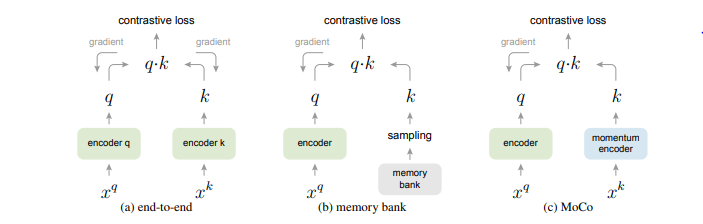Evolution to MoCo

A very well known contrastive learning network currently is SimCLR . The overall architecture of SimCLR is shown in the following picture.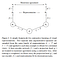SimCLR

The addition of a non-linear function g between loss and representation , f, is one key part of the architecture. As in other architectures, f is usually a ResNet, g is an MLP with ReLU . The authors also discovered that unsupervised contrastive learning benefits more from stronger data augmentation than supervised learning. The loss function, L[i,j], used in SimCLR is as follows.

L[i,j] = − log ( exp(sim(zi , zj )/τ ) /SUMk( II exp(sim(zi , zk)/τ ) ) )

Where sim(u, v) = Trans(u) v/|u||v|, denotes the dot product between L2 normalized u and v also known as cosine similarity). II is 1 iff k !=i, else 0. k ranges from 1 to 2N, N is the number of samples in the mini-batch.

One caveat of contrastive network is collapsed representations, where a representation is constant across different augmented views of the same image. In other words resulting in the same representation vector for all images. Collapsing is commonly avoided by using discriminating negative samples. However that increases training time as many negative samples need to be processed.

In the first paper, BYOL , “Bootstrap Your Own Latent — A New Approach to Self-Supervised Learning ”, the authors improve contrastive learning. BYOL does away with negative samples. The following figure shows BYOL with its 2 branches, called online and target. In the diagram, sg stands for stop gradient, θ and ξ are respectively weights of the online and target networks.

In BYOL, the target network differs from online network by the absence of a prediction network and uses of exponential average of weights of the online network. As in MoCo, f and g are ResNet and MLP respectively, q is also MLP. The loss used in BYOL is a mean squared error between the normalized predictions and target projections. The equations are shown in the following diagram. The loss is symmetricized by swapping the input sample pair.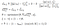Loss equations of BYOL

Input to BYOL is generated from a set of images D as follows. An image x of D is sampled uniformly from D, and two distributions of image augmentations T and T’, produces two augmented views v = t(x) and v’ = t’ (x) from x by applying respectively image augmentations t of T and t’ of T’ .

BYOL with a ResNet-200 (2× — width of hidden layers 2 times that of normal network), reaches a top-1 accuracy of 79.6%. Which is an improvement over the previous state of the art (76.8%) while uses 30% fewer parameters. However it is not intuitively clear how the predictor network and exponential weight average helps in avoiding representation collapse and achieve high accuracy.

The next paper is “Self-Supervised Visual Representation Learning from Hierarchical Grouping ”. The authors use a few non-DL computer vision techniques to generate per pixel positive and negative labels — Structured Edge Detector (SE) , Oriented Watershed Transform (OWT) , Ultrametric Contour Map (UCM) . SE uses ensemble of Random Forest algorithm, hence involves learning. SE has better accuracy than Canny edge and contour detector. OWT-UCM  combines OWT followed by UCM. OWT provides the initial regions that is used by UCM to provide segmentation by forming hierarchy of regions. Authors used the Berkeley Segmentation Dataset (BSDS) to train this part of the architecture.

In Watershed Transform (WT), an image is regarded as a topographic landscape with ridges and valleys in the landscape defined by some metric such as gray values of pixels. The watershed transform decomposes such 3D transform of an image into catchment basins. For each local minimum (P0), a catchment basin comprises all points whose path of steepest descent terminates at the minimum. Watersheds are the ridges/boundaries that separate basins from each other. The output is a set of contours of finest /smallest region of segmentation. Contours are lines that connect locations of equal value in any image.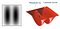Watershed of a synthetically generated of 2 blobs 

Ultrametric as the name implies is a generalized metric space, where the triangle inequality is strengthened. In metric space c =< a+b , where a,b and c are lengths of the sides of a triangle. Whereas in ultrametric space, c =< max(a,b). UCM generates a tree representing hierarchy of regions from contours by iteratively merging regions separated by the least weight boundaries.

The input to OWT is an image represented by E(x, y, θ). E(x, y, θ) is the output from SE and predicts the probability of an image boundary at location (x, y) and orientation θ. The output from OWT are a set of catchment basin minimum (P0), ridges/boundaries (K0), and weights as E(x, y, θ) at each point of K0 in the orientation of the approximate line segment. The line segments are linear approximation of contour arcs. Arcs are usually represented in a parametric form (x(u), y(u) ), where u is parameter that uniquely identifies a point in the arc. Hence there can be point to point correspondence between the arc and approximate line segment. The use of orientation of the approximate line segment is improvement of OWT over plain WT. Orientation enforces consistency between the strength of the boundaries of K0 and the underlying E(x, y, θ) signal, and removes artifacts of the WT.

UCM is a greedy graph algorithm. Input to UCM is a graph composed of P0 as vertices, K0 as edges, and E(x, y, θ) at each point of K0 as edge weight. The algorithm proceeds by sorting the edges by similarity and iteratively merging the most similar regions. This process produces a tree of regions, where the leaves are the elements of P0, the root is the entire image, and the regions are ordered by the inclusion relation.

Similarity between two adjacent regions is defined as the average strength of their common boundary in K0. The average strength does not decrease, or is monotonic, as larger and larger regions are merged. It is also guaranteed to produce an ultrametric distance on P0 × P0. The constructed region tree has the structure of an indexed hierarchy and can be described by a dendrogram tree. The height of each segment in the tree is the value of the similarity at which it first appears and the distance between two regions is the height of the smallest segment in the hierarchy containing them. The entire hierarchy can be represented as an Ultrametric Contour Map (UCM), where contour lines on the image are obtained by weighting each boundary between two regions by its scale of disappearance. UCM produces closed curves at any hierarchy. Thus, segmentation is easily obtained by thresholding at any level. The following picture shows the OWT and UCM algorithms.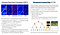OWT and UCM algorithms 

The following figure shows the high level block diagram of the full architecture used in the Hierarchical Grouping paper.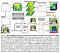Self-Supervised Visual Representation Learning from Hierarchical Grouping Architecture 

The architecture utilizes the tree of region hierarchy produced by OWT-UCM to provide ground truth per pixel. The distance between two regions is taken as the height of the sub-tree at which the two region disappear. The region distance is used in measuring the probability of a pixel j being similar to another pixel i. The paper limits the number of regions to 40 at the finest level of the region hierarchy tree.

The CNNs in the picture are randomly initialized ResNet50s. The 2 CNNs forms a Contrastive Learning. The feature vector is of hypercolumn design that combines feature maps coming from different blocks of ResNet. Specifically, 3×3 Conv-BN-ReLU block are used to project the last outputs of the Res3 and Res4 blocks into a 256-channel feature maps. The two feature maps are both interpolated to match the spatial resolution of a 4× downsampled input image before concatenation. The concatenated feature map is projected using a single 3 × 3 convolution layer, to produce a final 32-channel feature map as the output semantic embedding.

The distance between 2 pixels is defined as some average weight of the edge of 2 neighboring segments containing the pixels — d(S1,S2) = E(S1, S2) . The neighboring segments are found by traversing the region hierarchy tree just below the level where the regions merge into a single region. The following picture shows how the distance between non-neighboring regions , S2 and S4, is determined. This way the distance between any 2 pixels in the image can be determined.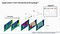Distance between pixels determined by traversing the region tree.

The region distance between 2 pixels is taken as a measure of probability they are part of the same segment. The following figure shows the equations for positive and negative probability. Optimization is then done to maximize and minimize the positive and negative probability for positive pairs and negative pairs respectively. The authors did not formulate P(j ∈ Neg(i)) as the complement of P(j ∈ P os(i)), to exclude pixels whose assignments are vague.Positive and negative probability of 2 pixels being in the same segment.

The input images are augmented by random resized cropping, random horizontal flipping, and color jittering, and then resized to 224 × 224. For each image, 7 regions are sampled and 10 positive pixels and 5 negative pixels are sampled from each sampled region.

The paper claims accuracy similar to MoCo at 200 epochs at just 80 epochs — 46.5 vs 47.01 mIoU of MoCo. At 80 epocs MoCo’s accuracy is 42.07.

The last paper, “Contrastive learning of global and local features for medical image segmentation with limited annotations ”, looks at MRI images. MRI and CT images comes in volumes composed of 2D slices. The authors proposed 2 changes to contrastive losses. One at global scale called Global Contrastive Loss (GCL) and the other at local scale (pixels or within a single 2D slice) called Local Contrastive Loss (LCL). The authors incorporate domain knowledge in selecting similar and dissimilar pair of images and pixels/regions. In the case of the paper , authors leverage the knowledge of the domain that volumetric images (MRI, CT) of same anatomical region of different subjects have similar content. Such images are also fairly aligned as produced by respective machines, hence requiring little or no registration corrections.

The authors divided each volume into partitions composed of consecutive 2D slices. The 2D images in a partition are considered similar for optimizing GCL . The following set of equations define GCL.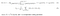Global Contrastive Loss

In the above equations, x˜ = t˜(x) and xˆ = tˆ(x) and t,˜ tˆ ∈ T , where T is the set of simple transformations such as crop followed by color transformations, etc. Λ+ is the set of all similar pairs of images that can be constructed from a given set of sampled images X from the volumes. Λ- is the set of pairs of dissimilar images. Both Λ+ and Λ- depends on the strategy used.

The authors used 3 different GCL loss strategies, each resulting in unique Λ+ and Λ- sets.

Random Strategy (GR) — where N slices from volumes are randomly selected. The set of similar pairs, Λ+, are pairs of transforms of the same image (x˜[i, s] , xˆ[ i, s]). The set of Λ- consists of pairs of the rest (N-1) images.

In strategy GD- , dissimilar images are restricted to dissimilar partitions.

Λ− = {x[ l, k] , x˜ [l, k] , xˆ[ l ,k] |∀k != s, ∀l}.

In strategy GD, set of similar images, Λ+, is expanded to include same partitions in other volumes — (x [i, s] , x[j, s] ), as well as transformed versions (x˜[ i, s] , xˆ[ j, s] ). Λ− is same as in GD- .

For LCL, features of each 2D slice is divided into regions, and pixels in a region are considered similar. Let ˜f and ˆf be the features of a pair of transform (˜x, xˆ) of an image x. Let the index of a region be denoted by (u, v). Corresponding local regions in ˜f and ˆf form the similar pair set Ω+. For each similar pair, the dissimilar set Ω− consists of all other local regions in both feature maps, ˜f and ˆf. The per mini-batch (X) LCL is defined by the following equations.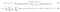Local Contrastive Loss on the feature map of a 2D slice.

The authors define Random Sampling (LR) strategy for LCL as choosing a 2D slice x[i,s] randomly and using ( ˜f [i,s](u, v), ˆf [i,s](u, v)) pairs for multiple s, i and (u, v) indices to form Ω +. For each similar pair, the dissimilar set Ω- consists of representations of all other local regions’ indices (u’ , v’ ) with u’ != u, v’ != v within the same feature maps ˜f [i ,s] and ˆf [i, s] — dissimilar regions from same slice. In the LD strategy, they extend LR strategy by adding to Ω+ corresponding local regions from differtent volumes ( ˜f [i,s](u, v), ˆf [j,s](u, v)). Ω− is composed of ˜f [i,s](u’, v’) and ˆf [j,s](u’, v’)), or dissimilar regions from slices of different volumes.

The authors used 3 datasets to explore their ideas as follows. ACDC dataset  — 100 3D cardiac cine-MRIs with 3 structures annotated — left ventricle, myocardium and right ventricle . Prostate dataset  — 48 3D prostate MRIs with 2 structures annotated, peripheral zone and central gland. MMWHS dataset  — 20 3D cardiac MRIs with annotations for 7 structures: left ventricle, left atrium, right ventricle, right atrium, myocardium, ascending aorta, and pulmonary artery.

The authors used UNet  based encoder-decoder network architecture. Encoder consists of 6 convolutional blocks, each consisting of two 3 × 3 convolutions followed by a 2 × 2 maxpooling layer with stride 2. The decoder is composed of a number of blocks. Each decoder block consists of one upsampling layer with a factor of 2, followed by concatenation from corresponding level of encoder via a skip connection, followed by two 3 × 3 convolutions.

The authors pre-train the encoder and decoder sub-networks separately. The encoder is trained with GCL. The decoder is trained with LCL. For decoder training, the decoder is divided in 2 parts. Only the first part with certain number of blocks is used during LCL pre-training. For fine-tuning, all the blocks of the decoder are used along with the encoder. The authors fine-tune the complete network to complete the overall training.

The authors found that their method reaches within 8% of benchmark (supervised) performance using only two labeled MRI volumes for training, corresponding to only 4% (for ACDC) of the training data used to train the benchmark.

References:

1. Bootstrap Your Own Latent — A New Approach to Self-Supervised Learning, arxiv:https://arxiv.org/pdf/2006.07733.pdf; nips: https://neurips.cc/virtual/2020/protected/poster_f3ada80d5c4ee70142b17b8192b2958e.html; code: https://github.com/deepmind/deepmind-research/tree/master/byol
2. Self-Supervised Visual Representation Learning from Hierarchical Grouping, arxiv: https://arxiv.org/pdf/2012.03044.pdf; nips: https://neurips.cc/virtual/2020/protected/poster_c1502ae5a4d514baec129f72948c266e.html
3. Contrastive learning of global and local features for medical image segmentation with limited annotations, nips:https://neurips.cc/virtual/2020/protected/poster_949686ecef4ee20a62d16b4a2d7ccca3.html; arxiv: https://arxiv.org/pdf/2006.10511.pdf; code:https://github.com/krishnabits001/domain_specific_cl
4. Revisiting Self-Supervised Visual Representation Learning, https://arxiv.org/pdf/1901.09005.pdf
5. Momentum Contrast for Unsupervised Visual Representation Learning, https://arxiv.org/pdf/1911.05722.pdf
6. A Simple Framework for Contrastive Learning of Visual Representations, https://arxiv.org/pdf/2002.05709.pdf
7. Dimensionality Reduction by Learning an Invariant Mapping, http://yann.lecun.com/exdb/publis/pdf/hadsell-chopra-lecun-06.pdf
8. Comparison of Faster-RCNN and Detection Transformer (DETR), https://whatdhack.medium.com/comparison-of-faster-rcnn-and-detection-transformer-detr-f67c2f5a2a04
9. Fast Edge Detection Using Structured Forests, https://arxiv.org/abs/1406.5549 .
10. The Watershed Transform: Strategies for Image Segmentation, https://www.mathworks.com/company/newsletters/articles/the-watershed-transform-strategies-for-image-segmentation.html
11. Boundary Extraction in Natural Images Using Ultrametric Contour Maps, https://citeseerx.ist.psu.edu/viewdoc/download?doi=10.1.1.123.9972&rep=rep1&type=pdf
12. From Contours to Regions: An Empirical Evaluation, paper:https://ttic.uchicago.edu/~mmaire/papers/pdf/amfm_cvpr2009_poster.pdf , poster: https://ttic.uchicago.edu/~mmaire/papers/pdf/amfm_cvpr2009_poster.pdf
13. ACDC dataset, Automated cardiac diagnosis challenge (acdc). https://www.creatis.insa-lyon.fr/Challenge/ acdc
14. Prostate dataset, Medical segmentation decathlon chalenge. http://medicaldecathlon.com/index.html
15. MMWHS dataset, Multi-modality whole heart segmentation challenge. http://www.sdspeople.fudan.edu. cn/zhuangxiahai/0/mmwhs .
16. U-net: Convolutional networks for biomedical image segmentation. In: International Conference on Medical image computing and computer-assisted intervention, https://link.springer.com/content/pdf/10.1007%2F978-3-319-24574-4_28.pdf